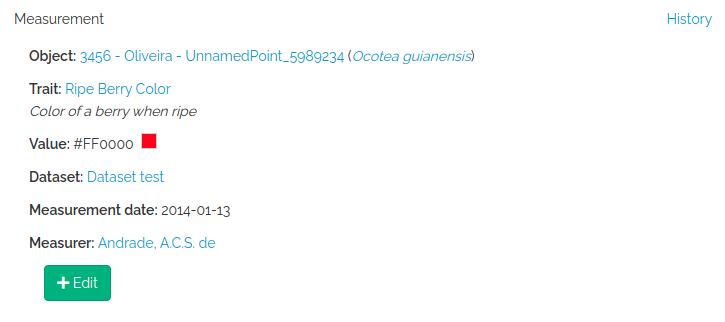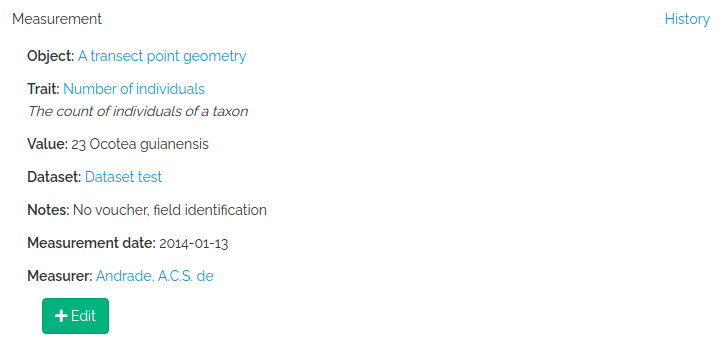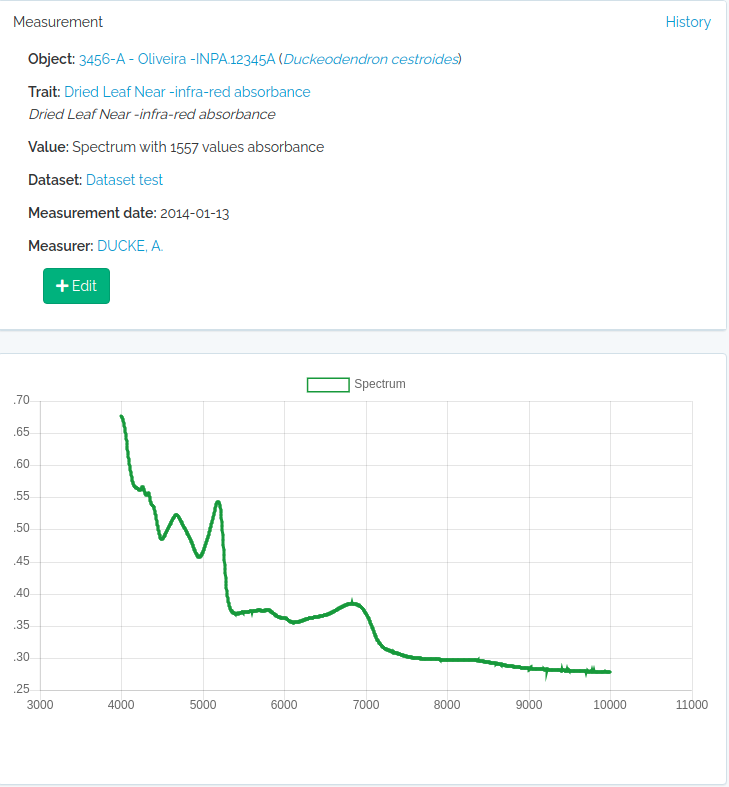# Import Measurements

Import Measurements using the OpenDataBio R client

Measurements can be imported using `odb_import_measurements()`. Read carefully the Measurements POST API.

## Quantitative measurements

``````library(opendatabio)
base_url="http://localhost:8080/api"
token ="GZ1iXcmRvIFQ" #this must be your token not this value
cfg = odb_config(base_url=base_url, token = token)

#get the trait id from the server (check that trait exists)
#generate some fake data for 10 measurements

dbhs = sample(seq(10,100,by=0.1),10)
object_ids = sample(1:3,length(dbhs),replace=T)
dates = sample(as.Date("2000-01-01"):as.Date("2000-03-31"),length(dbhs))
dates = lapply(dates,as.Date,origin="1970-01-01")
dates = lapply(dates,as.character)
dates = unlist(dates)

to.odb = data.frame(
trait_id = 'dbh',
value = dbhs,
date = dates,
object_type = 'Individual',
object_id=object_ids,
person="Oliveira, A.A. de",
dataset = 1,
notes = "some fake measurements",
stringsAsFactors=F)

#this will only work if the person exists, the individual ids exist
#and if the trait with export_name=dbh exist
odb_import_measurements(to.odb,odb_cfg=cfg)
``````

Get the imported data:

``````dad = odb_get_measurements(params = list(dataset=1),odb_cfg=cfg)
"measurementValue", "measurementUnit", "measurementDeterminedDate",
``````
``````id      basisOfRecord           measured_type measured_id measurementType measurementValue measurementUnit measurementDeterminedDate
1   1 MeasurementsOrFact App\\Models\\Individual           3             dbh             86.8     centimeters                2000-02-19
2   2 MeasurementsOrFact App\\Models\\Individual           2             dbh             84.8     centimeters                2000-03-25
3   3 MeasurementsOrFact App\\Models\\Individual           2             dbh             65.7     centimeters                2000-03-15
4   4 MeasurementsOrFact App\\Models\\Individual           3             dbh             88.0     centimeters                2000-03-05
5   5 MeasurementsOrFact App\\Models\\Individual           3             dbh             35.3     centimeters                2000-01-04
6   6 MeasurementsOrFact App\\Models\\Individual           2             dbh             36.0     centimeters                2000-03-23
7   7 MeasurementsOrFact App\\Models\\Individual           2             dbh             78.6     centimeters                2000-03-22
8   8 MeasurementsOrFact App\\Models\\Individual           2             dbh             69.7     centimeters                2000-03-09
9   9 MeasurementsOrFact App\\Models\\Individual           3             dbh             12.3     centimeters                2000-01-30
10 10 MeasurementsOrFact App\\Models\\Individual           3             dbh             14.7     centimeters                2000-01-18
1  Dataset test CC-BY 4.0
2  Dataset test CC-BY 4.0
3  Dataset test CC-BY 4.0
4  Dataset test CC-BY 4.0
5  Dataset test CC-BY 4.0
6  Dataset test CC-BY 4.0
7  Dataset test CC-BY 4.0
8  Dataset test CC-BY 4.0
9  Dataset test CC-BY 4.0
10 Dataset test CC-BY 4.0
``````

## Categorical measurements

Categories MUST be informed by their ids or name in the `value` field. For CATEGORICAL or ORDINAL traits, `value` must be single value. For CATEGORICAL_MULTIPLE, `value` may be one or multiple categories ids or names separated by one of `| or ; or ,`.

``````library(opendatabio)
base_url="http://localhost:8080/api"
token ="GZ1iXcmRvIFQ" #this must be your token not this value
cfg = odb_config(base_url=base_url, token = token)

#a categorical trait
(odbtraits = odb_get_traits(params=list(name="specimenFertility"),odb_cfg = cfg))

#base line
to.odb = data.frame(trait_id = odbtraits\$id, date = '2021-07-31', stringsAsFactors=F)

#the plant was collected with both flowers and fruits, so the value are the two categories
value = c("Flowers","Fruits")

#get categories for this trait if found
(cats = odbtraits\$categories[])
#check that your categories are registered for the trait and get their ids
value = cats[match(value,cats\$name),'id']
#make multiple categories ids a string value
value = paste(value,collapse=",")

to.odb\$value = value

to.odb\$object_type = "Voucher"

#get voucher id from API (must be ID).
#Search for collection number 1234
odbspecs = odb_get_vouchers(params=list(number="3456-A"),odb_cfg=cfg)
to.odb\$object_id = odbspecs\$id

#get dataset id
odbdatasets = odb_get_datasets(params=list(name='Dataset test'),odb_cfg=cfg)
to.odb\$dataset = odbdatasets\$id

#person that measured
odbperson = odb_get_persons(params=list(search='ana cristina sega'),odb_cfg=cfg)
to.odb\$person = odbperson\$id

#import'
odb_import_measurements(to.odb,odb_cfg=cfg)

#get imported
"measurementValue", "measurementUnit", "measurementDeterminedDate",
``````
``````id      basisOfRecord        measured_type measured_id   measurementType measurementValue measurementUnit
1 11 MeasurementsOrFact App\\Models\\Voucher           1 specimenFertility  Flowers, Fruits              NA
1                2021-07-31 Dataset test CC-BY 4.0
``````

## Color measurements

For color values you have to enter color as their `hex RGB strings` codes, so they can be rendered graphically and in the web interface. Therefore, any color value is allowed, and it would be easier to use the palette colors in the web interface to enter such measurements. Package `gplots` allows you to convert color names to hex RGB codes if you want to do it through the API.

``````library(opendatabio)
base_url="http://localhost:8080/api"
token ="GZ1iXcmRvIFQ" #this must be your token not this value
cfg = odb_config(base_url=base_url, token = token)

#get the trait id from the server (check that trait exists)
odbtraits = odb_get_traits(odb_cfg=cfg)
(m = match(c("fruitColor"),odbtraits\$export_name))

#base line
to.odb = data.frame(trait_id = odbtraits\$id[m], date = '2014-01-13', stringsAsFactors=F)

#get color value
#install.packages("gplots",dependencies = T)
library(gplots)
(value =  col2hex("red"))
to.odb\$value = value

to.odb\$object_type = "Individual"
#get voucher id from API (must be ID). Search for collection number 1234
odbind = odb_get_individuals(params=list(tag='3456'),odb_cfg=cfg)
odbind\$scientificName
to.odb\$object_id = odbind\$id

#get dataset id
odbdatasets = odb_get_datasets(params=list(name='Dataset test'),odb_cfg=cfg)
to.odb\$dataset = odbdatasets\$id

#person that measured
odbperson = odb_get_persons(params=list(search='ana cristina sega'),odb_cfg=cfg)
to.odb\$person = odbperson\$id

odb_import_measurements(to.odb,odb_cfg=cfg)
``````The LINK trait type allows one to register count data, as for example the number of individuals of a species at a particular location. You have to provide the linked object (`link_id`), which may be a Taxon or a Voucher depending on the trait definition, and then `value` recieves the numeric count.

``````library(opendatabio)
base_url="http://localhost:8080/api"
token ="GZ1iXcmRvIFQ" #this must be your token not this value
cfg = odb_config(base_url=base_url, token = token)

#get the trait id from the server (check that trait exists)
odbtraits = odb_get_traits(odb_cfg=cfg)
(m = match(c("taxonCount"),odbtraits\$export_name))

#base line
to.odb = data.frame(trait_id = odbtraits\$id[m], date = '2014-01-13', stringsAsFactors=F)

#the taxon to link the count value
odbtax = odb_get_taxons(params=list(name='Ocotea guianensis'),odb_cfg=cfg)

#now add the count value for this trait type
#this is optional for this measurement,
#however, it would make no sense to include such link without a count in this example
to.odb\$value = 23

#a note to clarify the measurement (optional)
to.odb\$notes = 'No voucher, field identification'

#this measurement will link to a location
to.odb\$object_type = "Location"
#get location id from API (must be ID).
#lets add this to a transect
to.odb\$object_id = odblocs\$id

#get dataset id
odbdatasets = odb_get_datasets(params=list(name='Dataset test'),odb_cfg=cfg)
to.odb\$dataset = odbdatasets\$id

#person that measured
odbperson = odb_get_persons(params=list(search='ana cristina sega'),odb_cfg=cfg)
to.odb\$person = odbperson\$id

odb_import_measurements(to.odb,odb_cfg=cfg)
``````## Spectral measurements

`value` must be a string of spectrum values separated by “;”. The number of concatenated values must match the Trait `value_length` attribute of the trait, which is extracted from the wavenumber range specification for the trait. So, you may easily check this before importing with `odb_get_traits(params=list(fields='all',type=9),cfg)`

``````library(opendatabio)
base_url="http://localhost:8080/api"
token ="GZ1iXcmRvIFQ" #this must be your token not this value
cfg = odb_config(base_url=base_url, token = token)

#second column are  NIR leaf absorbance values
#the spectrum has 1557 values
nrow(spectrum)
# 1557
#collapse to single string
value = paste(spectrum[,2],collapse = ";")
substr(value,1,100)
# "0.6768057;0.6763237;0.6755353;0.6746023;0.6733549;0.6718447;0.6701176;0.6682984;0.6662288;0.6636459;"

#get the trait id from the server (check that trait exists)
odbtraits = odb_get_traits(odb_cfg=cfg)
(m = match(c("driedLeafNirAbsorbance"),odbtraits\$export_name))

#see the trait
odbtraits[m,c("export_name", "unit", "range_min", "range_max",  "value_length")]
#export_name       unit range_min range_max value_length
#6 driedLeafNirAbsorbance absorbance   3999.64  10001.03         1557

#must be true
odbtraits\$value_length[m]==nrow(spectrum)
# TRUE

#base line
to.odb = data.frame(trait_id = odbtraits\$id[m], value=value, date = '2014-01-13', stringsAsFactors=F)

to.odb\$object_type = "Voucher"
#get voucher id from API (must be ID).
#search for a collection number
odbspecs = odb_get_vouchers(params=list(number="3456-A"),odb_cfg=cfg)
to.odb\$object_id = odbspecs\$id

#get dataset id
odbdatasets = odb_get_datasets(params=list(name='Dataset test'),odb_cfg=cfg)
to.odb\$dataset = odbdatasets\$id

#person that measuredJust add the text to the `value` field and proceed as for the other trait types.